# LaTex

Ir para: navegação, pesquisa

#### TeX and LaTeX (tirado de LaTeXPrimer)

TeX is a computer program for typesetting documents, created by D. E. Knuth. It takes a suitably prepared computer file and converts it to a form that may be printed on many kinds of printers, including dot-matrix printers, laser printers and high-resolution typesetting machines. A number of well-established publishers now use TeX in order to typeset books and mathematical journals.

Simple documents that do not contain mathematical formulae or tables may be produced very easily: the body of the text is typed in essentially unaltered (though observing certain rules regarding quotation marks and punctuation dashes). Typesetting mathematics is somewhat more involved, but even here TeX is comparatively straightforward to use when one considers the complexity of some of the formulae that it is required to typeset.

LaTeX, written by L. B. Lamport, is one of a number of dialects' of TeX. It is particularly suited to the production of long articles and books, since it has facilities for the automatic numbering of chapters, sections, theorems, equations etc., and also has facilities for cross-referencing. It is probably one of the most suitable version of LaTeX for beginners to use.

#### TeX e LaTeX (traduzido automaticamente por babelfish)

TeX é um programa de computador para typesetting originais, criado por D. E. Knuth. Faz exame de uma lima de computador apropriadamente preparada e converte-a a um formulário que possa ser imprimido em muitos tipos das impressoras, including impressoras da ponto-matriz, impressoras de laser e máquinas typesetting high-resolution. Um número de publishers well-established usam agora TeX a fim typeset livros e jornais matemáticos.

Os originais simples que não contêm fórmulas matemáticas ou tabelas podem ser produzidos muito fàcilmente: o corpo do texto é datilografado essencialmente em unaltered (though observando determinadas réguas a respeito das marcas da citação e dos traços da pontuação). A matemática typesetting é um tanto mais involvida, mas aqui TeX uniforme é comparativamente direto usar-se quando se considera a complexidade de algumas das fórmulas que se requer para typeset.

O laTeX, escrito por L. B. Lamport, é um de um número de dialects' do  de TeX. É servido particularmente à produção de artigos e livros longos, desde que têm facilidades para o numbering automático dos capítulos, seções, os theorems, as equações etc., e tem também facilidades para fazer remissão recíproca. É provavelmente uma da versão a mais apropriada do laTeX para novatos ao uso.

#### A Typical LaTeX Input File

The LaTeX program reads in text from a suitably prepared input file, and creates a DVI file' which encodes information on the fonts to be used and the positioning of the characters on the printed page. There are many programs available that can translate the DVI file' into page description languages such as PostScript', or convert it into the format appropriate for previewing the document on a computer screen or printing it out on dot-matrix printers.

Here is an example of a typical LaTeX input file:

\documentclass[a4paper,12pt]{article}
\begin{document}

The foundations of the rigorous study of \emph{analysis}
were laid in the nineteenth century, notably by the
mathematicians Cauchy and Weierstrass. Central to the
study of this subject are the formal definitions of
\emph{limits} and \emph{continuity}.

Let $D$ be a subset of $\bf R$ and let
$f \colon D \to \mathbf{R}$ be a real-valued function on
$D$. The function $f$ is said to be \emph{continuous} on
$D$ if, for all $\epsilon > 0$ and for all $x \in D$,
there exists some $\delta > 0$ (which may depend on $x$)
such that if $y \in D$ satisfies
$|y - x| < \delta$
then
$|f(y) - f(x)| < \epsilon.$

One may readily verify that if $f$ and $g$ are continuous
functions on $D$ then the functions $f+g$, $f-g$ and
$f.g$ are continuous. If in addition $g$ is everywhere
non-zero then $f/g$ is continuous.

\end{document}


When we apply LaTeX to these paragraphs we produce the text tex-tex-tex

This example illustrates various features of LaTeX. Note that the lines

\documentclass[a4paper,12pt]{article}
\begin{document}

are placed at the beginning of the input file.
These are followed by the main body of the text,
followed by the concluding line

\end{document}


Note also that, although most characters occurring in this file have their usual meaning, there are characters such as \, \$, { and } which have special meanings within LaTeX. In particular, there are sequences of characters which begin with a backslash' \ which are used to produce mathematical symbols and Greek letters and to accomplish tasks such as changing fonts. These are known as control sequences.

### Símbolos matemáticos

#### Letras gregas (minusculas)

\alpha   \beta  \gamma   \delta
\epsilon \zeta  \eta     \theta
\iota    \kappa \lambda  \mu
\nu      \xi    \pi      \rho
\sigma   \tau   \upsilon \phi
\chi     \psi   \omega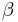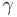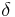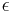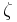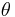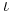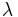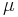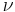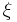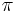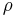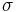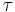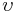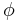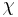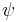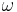#### Algumas tem variações

\varepsilon  \vartheta  \varpi
\varrho      \varsigma  \varphi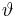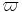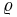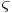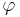#### Maiúsculas (nem todas tem)

\Gamma  \Delta    \Theta  \Lambda  \Xi     \Pi
\Sigma  \Upsilon  \Phi    \Psi     \Omega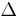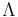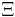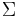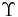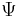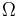### Figuras

Como controlar o lugar onde aparecem as figuras, isto é, como controlar o floating de figuras até forçar a barra, fixando elas onde queremos e não onde o LaTeX quer: